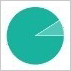# multiply

['mʌltɪplaɪ]['mʌltɪplaɪ]• 释义
• 用例
• 词组
• 讲解
• 更多
• vt. 乘；增加
• vi. 做乘法；繁衍### 释义常用度分布图

点击查看

双解释义
v. (动词)
1. vt. & vi. 增加 produce a large number of; make greater in number
2. vt. & vi. 繁殖 increase in number by procreation
3. vt. & vi. 乘 combine two numbers by adding one number to itself a certain number of times
详尽释义
1. 多样地
2. 多重地
3. 复合地
4. 多倍地
5. 复杂地
6. 【电】并联地
7. 多路地
1. 多股的
2. 多层的
v. (动词)
1. 增殖
2. 乘以
3. （使）成倍增加
4. 迅速增加
5. 【数】乘
6. 提出大量的…
7. 按比例放大
8. 增加
9. 做乘法
10. 繁殖
11. 使相乘
12. 使增加
13. 使繁殖
14. 相乘
15. （使）增多
16. 倍增
17. 扩大
18. 繁衍
英英释义
1. in several ways; in a multiple manner;

"they were multiply checked for errors"

Verb:
1. combine by multiplication;

"multiply 10 by 15"

2. combine or increase by multiplication;

"He managed to multiply his profits"

3. have young (animals) or reproduce (organisms);

"pandas rarely breed in captivity"
"These bacteria reproduce"

4. have offspring or produce more individuals of a given animal or plant;

"The Bible tells people to procreate"

• 例句
用作及物动词 (vt.)
1. If you multiply four by five, the answer is twenty.
四乘以五,答案是二十。
2. Let us multiply the height by the width to determine the area.
用宽乘以高来确定面积。
3. This will multiply our chances of success.
这将增加我们成功的机会。
4. He managed to multiply his profits.
他成功地增加了利润。
5. We have to multiply our efforts.
我们得更加努力。
用作不及物动词 (vi.)
1. He learned to multiply at the age of five.
他五岁学会做乘法。
2. The little boy can add, subtract and multiply, but cannot divide.
那小男孩会做加法、减法和乘法，但不会做除法。
3. Rabbits multiply quickly.
兔子繁殖很快。
4. Cells multiply by means of cell division.
细胞通过细胞分裂的方式来繁殖。
常见句型
用作动词 (v.)
用作不及物动词
S+～(+A)
1. Our chances of success multiplied.
我们成功的机会增加了。
2. Mistakes have been multiplying rapidly.
错误一直在快速增多。
3. Spending on military equipment has multiplied in the last five years.
过去5年中军事装备的开支大大增加。
4. Her anxieties multiplied until they could no longer be endured.
她的焦虑一直增加到不能再忍下去的程度。
5. Rabbits multiply rapidly.
兔子繁殖很快。
6. When animals have more food, they multiply faster.
当有更多的食物时,动物繁殖更快。
7. Can the boy multiply?
这男孩会做乘法吗?
用作及物动词
S+～+ n./pron.
1. Please multiply five and three.
把5和3相乘。
2. If you multiply 3 and 7, the answer is 21.3
和7相乘等于21。
3. This multiplied his chances of success.
这样做增多了他成功的机会。
4. He was asked to multiply instances.
人们要他举出更多的例证。
5. 1
6. The food of the dog has been multiplied.
那狗的食物增多了。
经典引文
• Till into seven it multiplies its stream.

• The activity of the emperor seemed to multiply his presence.

出自： Gibbon

• Thus truth was multiplied on truth.

出自： Tennyson

• Mental functioning is always multiply determined.

出自： J. Berman

• We were doubly, multiply, interlinked.

出自： S. Bellow

• 常用短语
用作动词 (v.)
multiply by (v.+prep.)
乘以 combine two numbers by adding one number to itself a certain number of times
multiply by sth
Three multiplied by four makes twelve. 3

乘以4得12。
Three multiplied by seven makes twenty-one. 3

乘以7等于21。 multiply sth by sth
Multiply the height by the width to determine the area.

长乘宽得面积。

增加…数量 increase the amount of sth
multiply sth ⇔ up
The costs have been multiplied up many times.

费用已增加了许多倍。

词汇搭配
用作动词 (v.)
～+副词 ～+介词
• 词语用法
v. (动词)
1. multiply的基本意思是“增加”,主要指通过自然繁殖而增加数量,也指同类事物在数量上无限制的重复增加。用于数学,可表示“相乘”。
2. multiply可用作及物动词,也可用作不及物动词。用作及物动词时,接名词或代词作宾语。
3. multiply作“相乘”解时常与介词by连用。
词义辨析
v. (动词)
multiply, breed, reproduce
• 这组词都有“繁殖”的意思。其区别在于:reproduce通常用于动物; breed用于人时通常含贬义; multiply强调大量地繁殖。
• 近反义词
【近义词】
【反义词】
临近单词